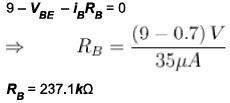Courses

# Bipolar Junction Transistor NAT Level -2

## 10 Questions MCQ Test Topic wise Tests for IIT JAM Physics | Bipolar Junction Transistor NAT Level -2

Description
This mock test of Bipolar Junction Transistor NAT Level -2 for Physics helps you for every Physics entrance exam. This contains 10 Multiple Choice Questions for Physics Bipolar Junction Transistor NAT Level -2 (mcq) to study with solutions a complete question bank. The solved questions answers in this Bipolar Junction Transistor NAT Level -2 quiz give you a good mix of easy questions and tough questions. Physics students definitely take this Bipolar Junction Transistor NAT Level -2 exercise for a better result in the exam. You can find other Bipolar Junction Transistor NAT Level -2 extra questions, long questions & short questions for Physics on EduRev as well by searching above.
*Answer can only contain numeric values
QUESTION: 1

### Calculate VCE in the figure (in volts).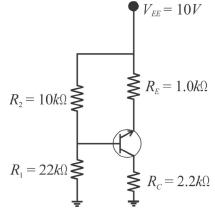Solution:

First check to see if Rin (Base) can be neglected.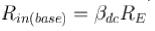=150 x kΩ = 150kΩ
Since 150kΩ is more than 10 times R2. The condition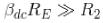is met and Rin(base) can be neglected.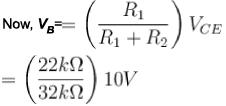= 6.88 V
Then, VE = VB + VBE
= 6.88 + 0.7
VE = 7.58V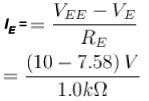lE = 2.42 mA
From IE we can determine lc and VCE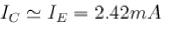and VC = ICRC = 2.42 mA x 2.2kΩ
= 5.32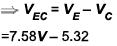= 2.26V

*Answer can only contain numeric values
QUESTION: 2

### Calculate the minimum value of C2 (in μF) if the amplifier operate over a frequency range of 2kHz to 10kHz?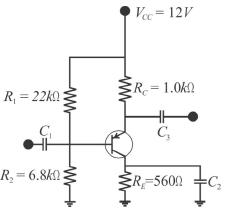Solution:

RE = 560Ω
XC of the bypass capacitor C2 should be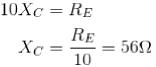The capacitive value, at the minimum frequency of 2kHz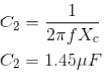*Answer can only contain numeric values
QUESTION: 3

### If the Q-point is 2 Vand 1 mA with the help of a feedback resistor RB. β = 100, then what is the value of RB in? (Take VBE = 0.7V).

Solution: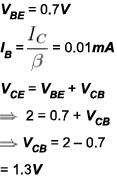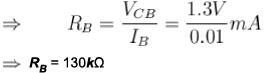*Answer can only contain numeric values
QUESTION: 4

Calculate the value of VCE (in Volts) for the circuit shown below. (β = 100 and VBE =0.7V).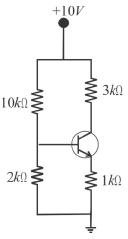Solution:

Let us use Thevenin’s theorem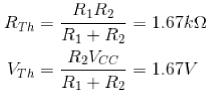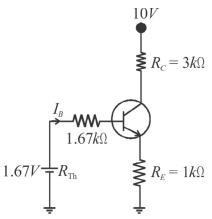Now, applying KVL to Base circuit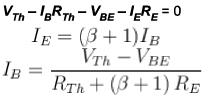= 9.45 μA
lC = 0.945mA
Now, Vcc = IcRc
- VCE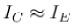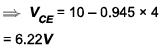*Answer can only contain numeric values
QUESTION: 5

A npn transistor circuit has  ∝ = 0.985. If IC = 2mA then the value of lB (in mA) is?

Solution: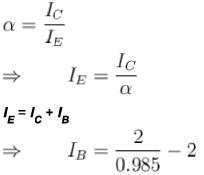= 0.03 mA

*Answer can only contain numeric values
QUESTION: 6

Calculate the collector voltage VC of the transistor circuit should be in the figure :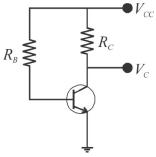(given = 0.96, lCBO = 20μA , VBE = 0.3V, RB = 100/kΩ)

Solution: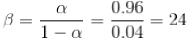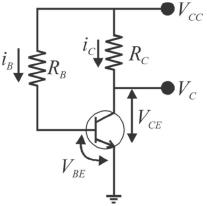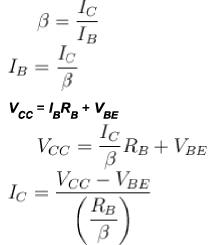Similarly,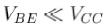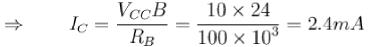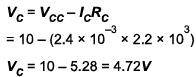*Answer can only contain numeric values
QUESTION: 7

In the below figure, the minimum value of lB (in mA) required to saturate this transistor if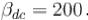[Neglect VCE(sat)].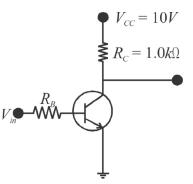Solution:

Since,VCE(Sat) is neglected (assume to 0V)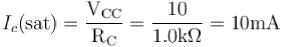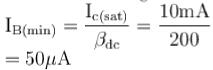*Answer can only contain numeric values
QUESTION: 8

In a certain oscillator, Av = 50. The attenuation of the feedback circuit must be?

Solution: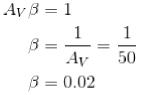*Answer can only contain numeric values
QUESTION: 9

An amplifier of gain 1000 is made into a feedback amplifier by feeding 9.9% of its output voltage in series with the input opposing. If fl = 20Hz and fh = 200kHz for the amplifier without feedback then due to the feedback what is the new frequency (in kHz)?

Solution: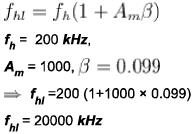*Answer can only contain numeric values
QUESTION: 10

In the transistor below, base current is 35 μA, VBE = 0.7V, what is the value of RB in kΩ?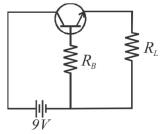Solution:

Using KVL,